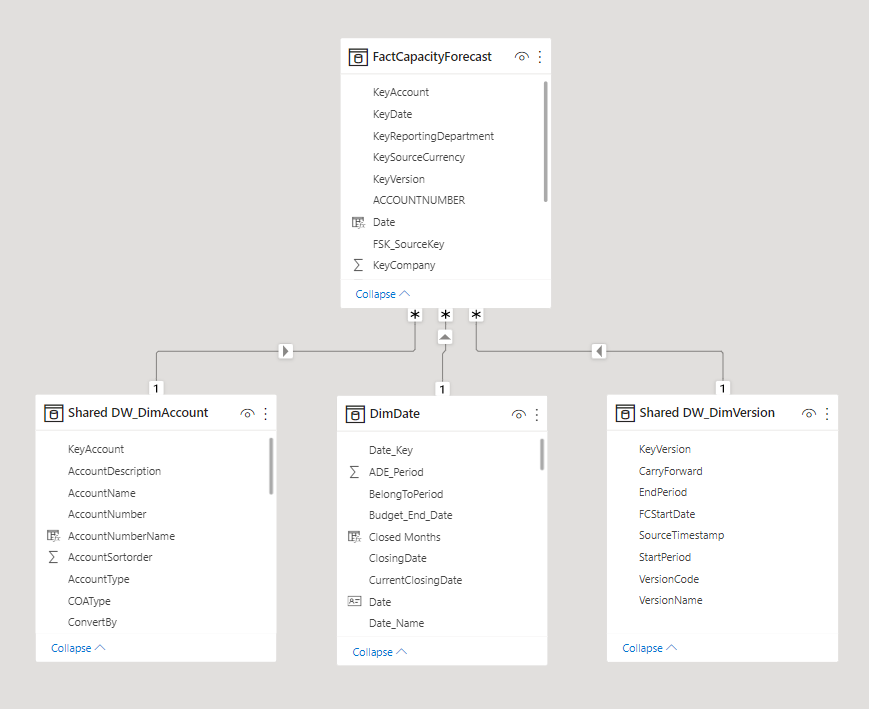cancel
Showing results for
Did you mean:

Fabric is Generally Available. Browse Fabric Presentations. Work towards your Fabric certification with the Cloud Skills Challenge.## Applying multiple filters on table (with the goal of avoiding repeated filters)

I have a report with slow visuals (to the point that it runs out of memory) and I am trying to optimize some of the DAX measures.

It is a big report, but the model for the part that I am having problems has 4 tables shown below:and the current measure is:

``````Capacity  = (CALCULATE(SUM(FactCapacityForecast[Value]),
FILTER('Shared DW_DimVersion', EDATE(MIN(DimDate[Date]),-1)='Shared DW_DimVersion'[FCStartDate]),
FILTER('Shared DW_DimAccount','Shared DW_DimAccount'[AccountNumber]="CALC0008"),
DATESBETWEEN(DimDate[Date],MIN(DimDate[Date]),EDATE(min(DimDate[Date]),5)))
+
CALCULATE(SUM(FactCapacityForecast[Value]),
FILTER('Shared DW_DimVersion', EDATE(MIN(DimDate[Date]),-1)='Shared DW_DimVersion'[FCStartDate]),
FILTER('Shared DW_DimAccount','Shared DW_DimAccount'[AccountNumber]="CALC0006"),
DATESBETWEEN(DimDate[Date],MIN(DimDate[Date]),EDATE(min(DimDate[Date]),5))))
*
(DIVIDE(
CALCULATE(SUM(FactCapacityForecast[Value]),
FILTER('Shared DW_DimVersion', EDATE(MIN(DimDate[Date]),-1)='Shared DW_DimVersion'[FCStartDate]),
FILTER('Shared DW_DimAccount','Shared DW_DimAccount'[AccountNumber]="CALC0017"),
DATESBETWEEN(DimDate[Date],MIN(DimDate[Date]),EDATE(min(DimDate[Date]),5))),
CALCULATE(SUM(FactCapacityForecast[Value]),
FILTER('Shared DW_DimVersion', EDATE(MIN(DimDate[Date]),-1)='Shared DW_DimVersion'[FCStartDate]),
FILTER('Shared DW_DimAccount','Shared DW_DimAccount'[AccountNumber]="CALC0016"),
DATESBETWEEN(DimDate[Date],MIN(DimDate[Date]),EDATE(min(DimDate[Date]),5)))))
*
(DIVIDE(
CALCULATE(SUM(FactCapacityForecast[Value]),
FILTER('Shared DW_DimVersion', EDATE(MIN(DimDate[Date]),-1)='Shared DW_DimVersion'[FCStartDate]),
FILTER('Shared DW_DimAccount','Shared DW_DimAccount'[AccountNumber]="CALC0005"),
DATESBETWEEN(DimDate[Date],MIN(DimDate[Date]),EDATE(min(DimDate[Date]),5))),
CALCULATE(SUM(FactCapacityForecast[Value]),
FILTER('Shared DW_DimVersion', EDATE(MIN(DimDate[Date]),-1)='Shared DW_DimVersion'[FCStartDate]),
FILTER('Shared DW_DimAccount','Shared DW_DimAccount'[AccountNumber]="CALC0006"),
DATESBETWEEN(DimDate[Date],MIN(DimDate[Date]),EDATE(min(DimDate[Date]),5))))*-1)*-1``````

I believe this can be written in a more efficient and readable way. Right now the filters are applied on 3 tables ('Shared DW_DimVersion', 'Shared DW_DimAccount' and 'DimDate') in each of CALCULATE functions and 2 of those are just being repeated. I want to create a table variable where I first apply filters on 'Shared DW_DimVersion' and 'DimDate' only once and then use that variable to apply filter on 'Shared DW_DimAccount' afterwards.

Maybe the final measure can be something like this:

``````Capacity Optimized =
VAR intermediate_table =
CALCULATETABLE(FactCapacityForecast,
//KEEPFILTERS(
FILTER(
//ALL ('Shared DW_DimVersion'[FCStartDate]),
'Shared DW_DimVersion',
EDATE(MIN('DimDate'[Date]),-1)='Shared DW_DimVersion'[FCStartDate]
)
//    )
,
KEEPFILTERS(    DATESBETWEEN(DimDate[Date],MIN(DimDate[Date]),EDATE(min(DimDate[Date]),5))
)
//DATESINPERIOD('DimDate'[Date],MIN('DimDate'[Date]), 5, MONTH)
)

RETURN
(CALCULATE(SUMX(intermediate_table, FactCapacityForecast[Value]),
KEEPFILTERS(
FILTER(
//ALL ('Shared DW_DimAccount'[AccountNumber]),
'Shared DW_DimAccount',
'Shared DW_DimAccount'[AccountNumber] = "CALC0008"
)
)
)
+
CALCULATE(SUMX(intermediate_table, FactCapacityForecast[Value]),
KEEPFILTERS(
FILTER(
//ALL ('Shared DW_DimAccount'[AccountNumber]),
'Shared DW_DimAccount',
'Shared DW_DimAccount'[AccountNumber] = "CALC0006"
)
)
))
*
DIVIDE(
CALCULATE(SUMX(intermediate_table, FactCapacityForecast[Value]),
KEEPFILTERS(
FILTER(
//ALL ('Shared DW_DimAccount'[AccountNumber]),
'Shared DW_DimAccount',
'Shared DW_DimAccount'[AccountNumber]  = "CALC0017"
)
)
),
CALCULATE(SUMX(intermediate_table, FactCapacityForecast[Value]),
KEEPFILTERS(
FILTER(
//ALL ('Shared DW_DimAccount'[AccountNumber]),
'Shared DW_DimAccount',
'Shared DW_DimAccount'[AccountNumber] = "CALC0016"
)
)
)
)
*
(DIVIDE(
CALCULATE(SUMX(intermediate_table, FactCapacityForecast[Value]),
KEEPFILTERS(
FILTER(
ALL ('Shared DW_DimAccount'[AccountNumber]),
'Shared DW_DimAccount'[AccountNumber] = "CALC0005"
)
)
),
CALCULATE(SUMX(intermediate_table, FactCapacityForecast[Value]),
KEEPFILTERS(
FILTER(
ALL ('Shared DW_DimAccount'[AccountNumber]),
'Shared DW_DimAccount'[AccountNumber] = "CALC0006"
)
)
)
)*-1)*-1``````

The results are supposed to be used in a matrix alongside 'DimDate[Month]' values.
I have been playing around with different functions, but cannot get the same number as the original measure.
How can I get the correct measure?

Thanks!
Moe

@OwenAuger I think you have a good solution for this problem as well 😄

1 ACCEPTED SOLUTIONSuper User

Hi @MoeData

``````Capacity =
VAR MinDate =
MIN ( DimDate[Date] )
VAR DimVersion =
FILTER (
'Shared DW_DimVersion',
'Shared DW_DimVersion'[FCStartDate] = EDATE ( MinDate, -1 )
)
VAR Dates =
DATESBETWEEN ( DimDate[Date], MinDate, EDATE ( MinDate, 5 ) )
VAR FactCapacity =
CALCULATETABLE ( FactCapacityForecast, DimVersion, Dates )
VAR CALC0005 =
CALCULATE (
SUM ( FactCapacityForecast[Value] ),
FactCapacity,
'Shared DW_DimAccount'[AccountNumber] = "CALC0005"
)
VAR CALC0006 =
CALCULATE (
SUM ( FactCapacityForecast[Value] ),
FactCapacity,
'Shared DW_DimAccount'[AccountNumber] = "CALC0006"
)
VAR CALC0008 =
CALCULATE (
SUM ( FactCapacityForecast[Value] ),
FactCapacity,
'Shared DW_DimAccount'[AccountNumber] = "CALC0008"
)
VAR CALC0016 =
CALCULATE (
SUM ( FactCapacityForecast[Value] ),
FactCapacity,
'Shared DW_DimAccount'[AccountNumber] = "CALC0016"
)
VAR CALC0017 =
CALCULATE (
SUM ( FactCapacityForecast[Value] ),
FactCapacity,
'Shared DW_DimAccount'[AccountNumber] = "CALC0017"
)
RETURN
( CALC0008 + CALC0006 )
* ( DIVIDE ( CALC0017, CALC0016 ) )
* ( DIVIDE ( CALC0005, CALC0006 ) * -1 ) * -1``````

2 REPLIES 2@tamerj1 Amazing! Thanks! Runs faster and much more readable.Super User

Hi @MoeData

``````Capacity =
VAR MinDate =
MIN ( DimDate[Date] )
VAR DimVersion =
FILTER (
'Shared DW_DimVersion',
'Shared DW_DimVersion'[FCStartDate] = EDATE ( MinDate, -1 )
)
VAR Dates =
DATESBETWEEN ( DimDate[Date], MinDate, EDATE ( MinDate, 5 ) )
VAR FactCapacity =
CALCULATETABLE ( FactCapacityForecast, DimVersion, Dates )
VAR CALC0005 =
CALCULATE (
SUM ( FactCapacityForecast[Value] ),
FactCapacity,
'Shared DW_DimAccount'[AccountNumber] = "CALC0005"
)
VAR CALC0006 =
CALCULATE (
SUM ( FactCapacityForecast[Value] ),
FactCapacity,
'Shared DW_DimAccount'[AccountNumber] = "CALC0006"
)
VAR CALC0008 =
CALCULATE (
SUM ( FactCapacityForecast[Value] ),
FactCapacity,
'Shared DW_DimAccount'[AccountNumber] = "CALC0008"
)
VAR CALC0016 =
CALCULATE (
SUM ( FactCapacityForecast[Value] ),
FactCapacity,
'Shared DW_DimAccount'[AccountNumber] = "CALC0016"
)
VAR CALC0017 =
CALCULATE (
SUM ( FactCapacityForecast[Value] ),
FactCapacity,
'Shared DW_DimAccount'[AccountNumber] = "CALC0017"
)
RETURN
( CALC0008 + CALC0006 )
* ( DIVIDE ( CALC0017, CALC0016 ) )
* ( DIVIDE ( CALC0005, CALC0006 ) * -1 ) * -1``````Announcements#### Power BI Monthly Update - November 2023

Check out the November 2023 Power BI update to learn about new features.#### Fabric Community News unified experience

Read the latest Fabric Community announcements, including updates on Power BI, Synapse, Data Factory and Data Activator.#### The largest Power BI and Fabric virtual conference

130+ sessions, 130+ speakers, Product managers, MVPs, and experts. All about Power BI and Fabric. Attend online or watch the recordings.Top Solution Authors
Top Kudoed Authors
Users online (4,923)